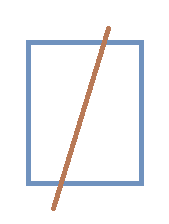# Division of Square

Geometry Level 4Let a straight line cuts a unit square in two parts such that ratio of their areas is 2:1. This line also passes through the midpoint of one of the intersecting sides of the square. Then find the intercepted length of the line within the square.

If the length comes in the form of $\dfrac{\sqrt{a}}{b}$, where $a$ and $b$ are positive integers and $a$ is square-free, then submit your answer as $a+b$.

×

Problem Loading...

Note Loading...

Set Loading...